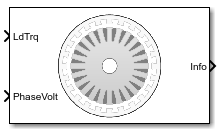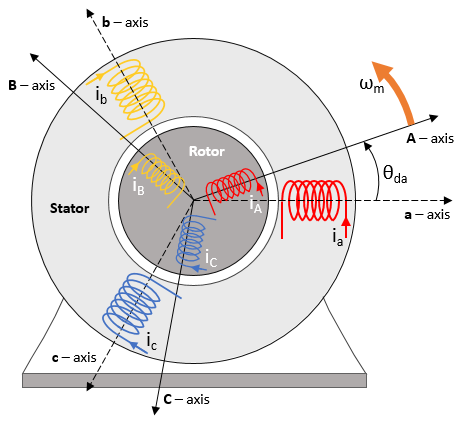# Induction Motor

Three-phase induction motor

• Library:
• Powertrain Blockset / Propulsion / Electric Motors

•## Description

The Induction Motor block implements a three-phase induction motor. The block uses the three-phase input voltages to regulate the individual phase currents, allowing control of the motor torque or speed.

By default, the block sets the Simulation Type parameter to `Continuous` to use a continuous sample time during simulation. If you want to generate code for fixed-step double- and single-precision targets, considering setting the parameter to `Discrete`. Then specify a Sample Time, Ts parameter.

### Three-Phase Sinusoidal Model Electrical System

The block implements equations that are expressed in a stationary rotor reference (qd) frame. The d-axis aligns with the a-axis. All quantities in the rotor reference frame are referred to the stator.The block uses these equations to calculate the electrical speed (ωem) and slip speed (ωslip).

To calculate the dq rotor electrical speed with respect to the rotor A-axis (dA), the block uses the difference between the stator a-axis (da) speed and slip speed:

To simplify the equations for the flux, voltage, and current transformations, the block uses a stationary reference frame:

`$\begin{array}{l}{\omega }_{da}=0\\ {\omega }_{dA}=-{\omega }_{em}\end{array}$`

CalculationEquation
Flux

Current

Inductance

Electromagnetic torque

Power invariant dq transformation to ensure that the dq and three phase powers are equal

The equations use these variables.

 ωm Angular velocity of the rotor ωem Electrical rotor speed ωslip Electrical rotor slip speed ωsyn Synchronous rotor speed ωda dq stator electrical speed with respect to the rotor a-axis ωdA dq stator electrical speed with respect to the rotor A-axis Θda dq stator electrical angle with respect to the rotor a-axis ΘdA dq stator electrical angle with respect to the rotor A-axis Lq, Ld q- and d-axis inductances Ls Stator inductance Lr Rotor inductance Lm Magnetizing inductance Lls Stator leakage inductance Llr Rotor leakage inductance vsq, vsd Stator q- and d-axis voltages isq, isd Stator q- and d-axis currents λsq, λsd Stator q- and d-axis flux irq, ird Rotor q- and d-axis currents λrq, λrd Rotor q- and d-axis flux va, vb, vc Stator voltage phases a, b, c ia, ib, ic Stator currents phases a, b, c Rs Resistance of the stator windings Rr Resistance of the rotor windings P Number of pole pairs Te Electromagnetic torque

### Mechanical System

The motor angular velocity is given by:

`$\begin{array}{c}\frac{d}{dt}{\omega }_{m}=\frac{1}{J}\left({T}_{e}-{T}_{f}-F{\omega }_{m}-{T}_{m}\right)\\ \frac{d{\theta }_{m}}{dt}={\omega }_{m}\end{array}$`

The equations use these variables.

 J Combined inertia of motor and load F Combined viscous friction of motor and load θm Motor mechanical angular position Tm Motor shaft torque Te Electromagnetic torque Tf Motor shaft static friction torque ωm Angular mechanical velocity of the motor

### Power Accounting

For the power accounting, the block implements these equations.

Bus Signal DescriptionVariableEquations

`PwrInfo`

`PwrTrnsfrd` — Power transferred between blocks

• Positive signals indicate flow into block

• Negative signals indicate flow out of block

`PwrMtr`

Mechanical power

Pmot

`PwrBus`

Electrical power

Pbus

`PwrNotTrnsfrd` — Power crossing the block boundary, but not transferred

• Positive signals indicate an input

• Negative signals indicate a loss

`PwrElecLoss`

Resistive power loss

Pelec

`PwrMechLoss`

Mechanical power loss

Pmech

When Port Configuration is set to `Torque`:

When Port Configuration is set to `Speed`:

`PwrStored` — Stored energy rate of change

• Positive signals indicate an increase

• Negative signals indicate a decrease

`PwrMtrStored`

Stored motor power

Pstr

The equations use these variables.

 Rs Stator resistance Rr Motor resistance ia, ib, ic Stator phase a, b, and c current isq, isd Stator q- and d-axis currents van, vbn, vcn Stator phase a, b, and c voltage ωm Angular mechanical velocity of the rotor F Combined motor and load viscous damping Te Electromagnetic torque Tf Combined motor and load friction torque

## Ports

### Input

expand all

Rotor shaft input torque, Tm, in N·m.

#### Dependencies

To create this port, select `Torque` for the Port configuration parameter.

Angular velocity of the rotor, ωm, in rad/s.

#### Dependencies

To create this port, select `Speed` for the Port configuration parameter.

Stator terminal voltages, Va, Vb, and Vc, in V.

### Output

expand all

The bus signal contains these block calculations.

Signal DescriptionVariableUnits

`IaStator`

Stator phase current A

ia

A

`IbStator`

Stator phase current B

ib

A

`IcStator`

Stator phase current C

ic

A

`IdSync`

Direct axis current

id

A

`IqSync`

iq

A

`VdSync`

Direct axis voltage

vd

V

`VqSync`

vq

V

`MtrSpd`

Angular mechanical velocity of the rotor

ωm

`MtrPos`

Rotor mechanical angular position

θm

`MtrTrq`

Electromagnetic torque

Te

N·m

`PwrInfo`

`PwrTrnsfrd`

`PwrMtr`

Mechanical power

Pmot

W
`PwrBus`

Electrical power

Pbus

W

`PwrNotTrnsfrd`

`PwrElecLoss`

Resistive power loss

Pelec

W
`PwrMechLoss`

Mechanical power loss

Pmech

W

`PwrStored`

`PwrMtrStored`

Stored motor power

Pstr

W

Phase a, b, c current, ia, ib, and ic, in A.

Motor torque, Tmtr, in N·m.

#### Dependencies

To create this port, select `Speed` for the Port configuration parameter.

Angular speed of the motor, ωmtr, in rad/s.

#### Dependencies

To create this port, select `Torque` for the Port configuration parameter.

## Parameters

expand all

#### Block Options

By default, the block uses a continuous sample time during simulation. If you want to generate code for single-precision targets, considering setting the parameter to `Discrete`.

#### Dependencies

Setting Simulation Type to `Discrete` creates the Sample Time, Ts parameter.

Integration sample time for discrete simulation, in s.

#### Dependencies

Setting Simulation Type to `Discrete` creates the Sample Time, Ts parameter.

This table summarizes the port configurations.

Port ConfigurationCreates Input PortCreates Output Port

`Torque`

`LdTrq`

`MtrSpd`

`Speed`

`Spd`

`MtrTrq`

Stator resistance, RS, in ohms and leakage inductance, Lls, in H.

Rotor resistance, Rr, in ohms and leakage inductance, Llr, in H.

Magnetizing inductance, Lm, in H.

Motor pole pairs, P.

Initial rotor angular position, θm0, in rad.

Initial angular velocity of the rotor, ωm0, in rad/s.

#### Dependencies

To enable this parameter, select `Torque` for the Port configuration.

Mechanical properties of the rotor:

• Inertia, J, in kg·m^2

• Viscous damping, F, in N·m/(rad/s)

• Static friction, Tf, in N·m

#### Dependencies

To enable this parameter, select `Torque` for the Port configuration.

 Mohan, Ned. Advanced Electric Drives: Analysis, Control and Modeling Using Simulink. Minneapolis, MN: MNPERE, 2001.

##### SupportGet trial now